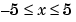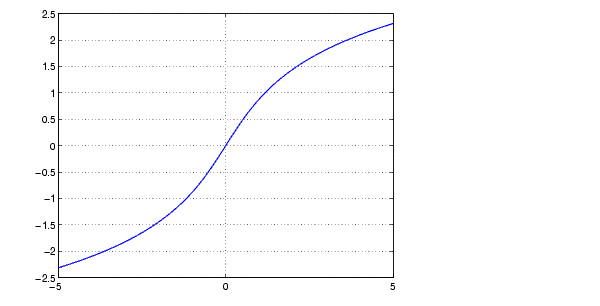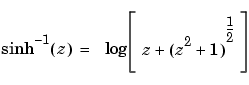MATLAB Function Referenceasinh

Inverse hyperbolic sine

Syntax

• ```Y = asinh(X)
```

Description

```Y = asinh(X) ``` returns the inverse hyperbolic sine for each element of `X`.

The `asinh` function operates element-wise on arrays. The function's domains and ranges include complex values. All angles are in radians.

Examples

Graph the inverse hyperbolic sine function over the domain.

• ````x = `-5:.01:5;
plot(x,asinh(x)), grid on
```Definition

The hyperbolic inverse sine can be defined as

•Algorithm

`asinh` uses FDLIBM, which was developed at SunSoft, a Sun Microsystems, Inc. business, by Kwok C. Ng, and others. For information about FDLIBM, see http://www.netlib.org.

See Also

`asin`, `sinh`

© 1994-2005 The MathWorks, Inc.